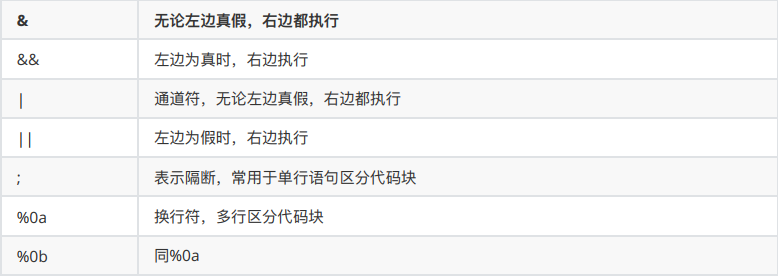# 命令执行漏洞

#### 简介

``````用户输入未过滤或净化（净化就是对特殊字符做处理如转义，然后再执行命令）

#### PHP下命令执行函数

##### 代码执行函数
``````eval()
assert()``````
##### 执行系统命令的函数
``````system：执行一个外部的应用程序并显示输出的结果
exec：执行一个外部的应用程序
shell_exec：执行shell命令并返回输出的结果的字符串
passthru：执行一个UNIX系统命令并显示原始的输出
``：与shell_exec函数的功能相同
popen：与shell_exec函数功能类似，popen(‘[系统命令]’, ‘r’)，’r’表示返回stdout文件指针，’w’表示返回stdin文件指针
proc_popen
pcntl_exec
\$(xxx)：在bash中用来做命令替换的，可以当做shell命令执行，但不是所有shell都支持``````
``````<?php
\$handle = popen('/path/to/executable 2>&1', 'r');
echo "'\$handle'; " . gettype(\$handle) . "\n";
pclose(\$handle);
?>``````

#### 命令拼接符``````command1 && command2 先执⾏command1，成功后在执⾏command2,如果失败则不执⾏command2
command1 & command2 先执⾏command1，⽆论成功与否都执⾏command2
command1 | command2 管道，直接将command1的输出作为command2的标准输⼊，只打印command2的标准

command1 || command2 或者， command1执⾏失败后执⾏command2,如果执⾏成功则不执⾏command2
command1 ; command2 前后之间没有关系，所有命令⽆论失败都会执⾏，从左往右依次执⾏``````

#### PHP函数

##### eval()

eval()函数会把字符串按照PHP代码来计算，该字符串必须是合法的PHP代码，且必须以分号结尾

``````<?php
eval("echo hello;");
?> ``````
##### assert()

assert()函数会将字符串当PHP代码来计算，不用是严格的PHP代码，且支持动态嵌套

``````\$a = "ass";
\$b = "ert";
\$c=\$a.\$b;
\$c(phpinfo(););

<?php
if(isset(\$_GET['code'])){
\$code=\$_GET['code'];
assert(\$code);
}else{
}
?>``````
##### call_user_func ()

call_user_func()等函数都有调⽤其他函数的功能,其中的⼀个参数作为要调⽤的函数名 ，该函数有两个参数,第⼀个参数作为回调函数,后⾯的参数作为回调函数的参数

``````<?php
if(isset(\$_GET['fun'])){
\$fun=\$_GET['fun'];
\$para=\$_GET['para'];
call_user_func(\$fun,\$para); call_user_func(assert,phpinfo(););
}else{
echo "?fun=assert&para=phpinfo()";
}
?>``````
##### 动态函数 a(b)

``````<?php
if(isset(\$_GET['a'])){
\$a=\$_GET['a'];
\$b=\$_GET['b'];
\$a(\$b); // assert(phpinfo();)
}else{
echo "
?a=assert&b=phpinfo()
";
}
?>``````
##### preg_replace ()

``````preg_replace(mixed \$pattern,mixed \$replacement, mixed \$subject [,int limit = -1[,int &\$count]])
/*
\$pattern: 要搜索的模式，可以是字符串或一个字符串数组
\$replacement: 用于替换的字符串或字符串数组。
\$subject: 要搜索替换的目标字符串或字符串数组。
\$limit: 可选，对于每个模式用于每个 subject 字符串的最大可替换次数。 默认是-1（无限制）。
\$count: 可选，为替换执行的次数。
*/
//典型代码如下:
<?php
if(isset(\$_GET['code'])){
\$code=\$_GET['code'];
preg_replace("/\((.*)\)/e", '\\1', \$code); // \\1 标识第⼀次匹配的内容 [.*]
}else{
echo "?code=[phpinfo()]";
}
?>

/(.*)/e
(phpinfo();)
(phpinfo();
?code=()
// 提交?code=(phpinfo();, phpinfo会被执⾏
(phpinfo();)``````

Daniel_WRF